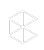Learn from the best and brightest in legal, technology, and beyond! Join us at the 2019 Clio Cloud Conference — San Diego | October 21-22, 2019.

How Is the Billable Hours Target Calculated?

Objective:

• What are the hours for the Billable Hours Target?
• How is the day/week/month/year Billable Hours calculated?

Environment:

• Clio Manage

The Billable Hours Target is calculated based a 7-day week (365 day year) by default, but this can be changed. Performance Metrics are based on a 5-day work week (260 day year).

The formula for calculating the Billable Hours Target is as follows:

We first divide the billable target for the entire year (target billings) by the number of working days in year to get the \$/day. We then divide that number by Default Billing Rate to get the number of hours per day. Range for monthly is 30, for yearly is 365 (by default, although you can change it) and for daily is 1.

We can simplify the equation as follows:

(Billable Target) * (Range) /(Number of working days in a year * Default Billing Rate) = Billable Hours Target by Duration

Example:

Find the Monthly Billable Hours Target when the firm's Billable Target is \$100,000 and Default Billing Rate is \$200/HR. Given that the number of working days in a year is 365.

Solution:

(100,000*30)/(365*200) = 41.1 Hours/Month